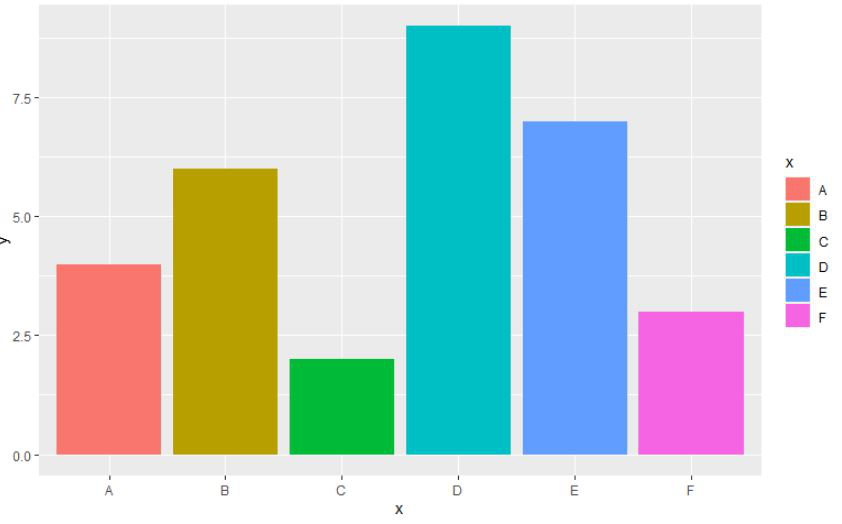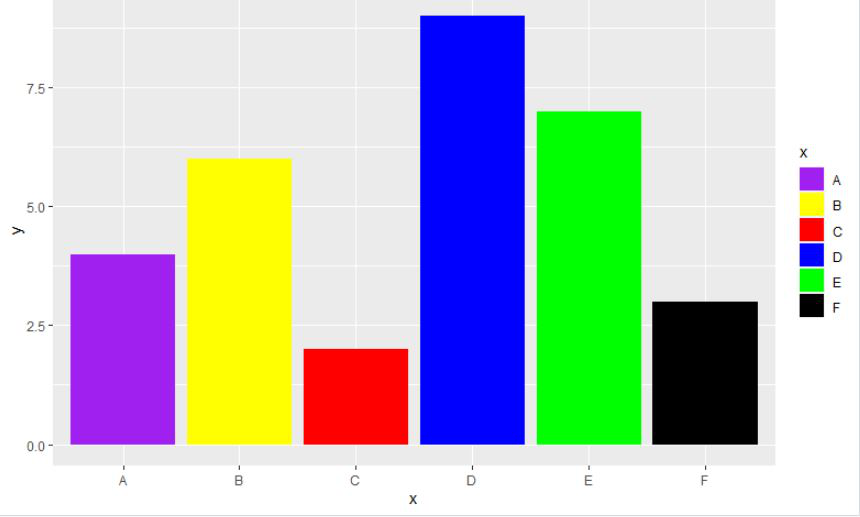Coloring Barplots with ggplot2 in R

• Last Updated : 24 Oct, 2021

In this article, we will discuss how to color the barplot using the ggplot2 package in the R programming language.

Method 1: Using fill argument within the aes function

Using the fill argument within the aes function to be equal to the grouping variable of the given data. Aesthetic mappings describe how variables in the data are mapped to visual properties (aesthetics) of geoms. Aesthetic mappings can be set in ggplot() and in individual layers

Syntax:

aes(x, y, ...)

Parameters:

x, y, … : List of name-value pairs in the form aesthetic = variable describing which variables in the layer data should be mapped to which aesthetics used by the paired geom/stat.

Example:

We will be using 6 different data points for the bar plot and then with the help of the fill argument within the aes function, we will be applying the default colors to the barplot in the R programming language.

R

 # load the librarylibrary("ggplot2")  # create the dataframe with letters and numbersgfg < -data.frame(    x=c('A', 'B', 'C', 'D', 'E', 'F'),    y=c(4, 6, 2, 9, 7, 3))  # display the barggplot(gfg, aes(x, y, fill=x)) + geom_bar(stat="identity")

Output:-Method 2: Using scale_fill_manual function

scale_fill_manual() allows you to specify your own set of mappings from levels in the data to aesthetic values.

Syntax:

scale_fill_manual(..., values)

Parameters:

…: Common discrete scale parameters: name, breaks, labels, na.value, limits, and guide.

Example:

We will be using 6 different data points for the bar plot and then with the help of using the scale_fill_manual function, we will be applying the given colors to the barplot in the R programming language.

Input:

R

 # load the packagelibrary("ggplot2")  # create a dataframe# with letters and numbersgfg < -data.frame(    x=c('A', 'B', 'C', 'D', 'E', 'F'),    y=c(4, 6, 2, 9, 7, 3))  # display barggplot(gfg, aes(x, y, fill=x)) +geom_bar(stat="identity") +scale_fill_manual(values=c("A"="purple",                           "B"="yellow",                           "C"="red",                           "D"="blue",                           "E"="green",                           "F"="black"))

Output:-My Personal Notes arrow_drop_up